# Pressure due to two liquids in a U-tube

brotherbobby
Homework Statement:
The figure below shows four situations in which a red liquid and a gray liquid are in a U-tube. In one situation the liquids cannot be in static equilibrium. (a) Which situation is that? (b) For the other three situations, assume static equilibrium. For each of them, is the density of the red liquid greater than, less than, or equal to the density of the gray liquid?
Relevant Equations:
(Gauge) pressure due to a liquid at a depth ##h## below the surface : ##P_G = \rho_L gh## where ##\rho_L## is the density of the liquid.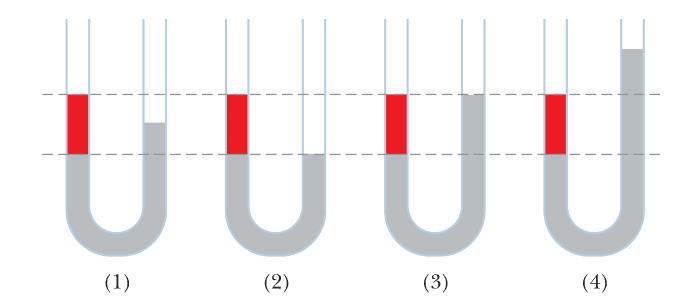(a) Situation (2) is my answer (which cannot be in static equilibrium). The pressures in the lower dashed line (##P_2##) has to be the same on either side (for equilibrium). That means the pressure due to the red liquid is equal to the pressure due to the same length of air column (length), which is not possible. I explain a bit in the cropped diagram shown below. ##P_A## = Pressure due to air and ##\color{red}{P_{LR}}## = Pressure due to red liquid.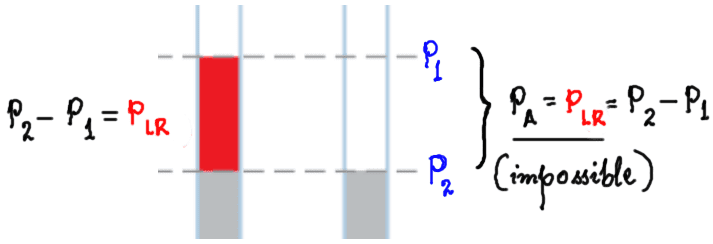(b) In situation 1, I begin by drawing on it.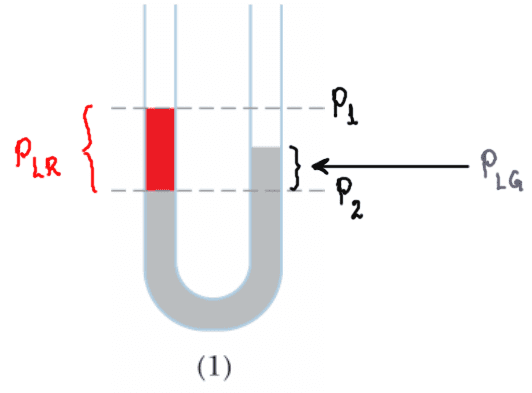We can see that the pressure ##P_2 = P_{\text{atm}} + \color{red}{P_{LR}} = P_{\text{atm}} + \color{grey}{ P_{LG}}\Rightarrow \color{red}{P_{LR}} =\color{grey}{ P_{LG}}##. Clearly since the height of the grey column is less than the red, equal pressures mean ##\boxed{\color{red} {\rho_{LR}} < \color{grey}{\rho_{LG}}}##. (You will note that I have ignored the little column of air by which the grey liquid is below the red liquid. I am assuming the pressure of air to be small compared to those due to the liquids. Or, we could add more grey liquid on the right side so that it's height is infinitesimally less than that of the red liquid which would cancel the pressure due to air on both sides exactly.)

Situation 3 is shown alongside.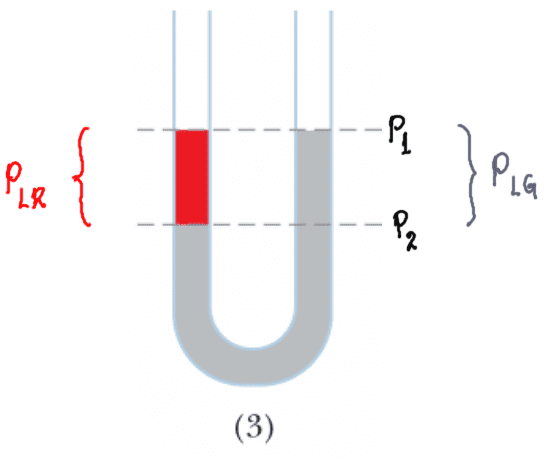Arguments are the same as before, focussing on equality of pressure ##P_2## on both sides which imply again that ##\color{red}{P_{LR}} = \color{grey}{ P_{LG}}##. But in this case since the liquid colums have the same heights, we conclude that ##\boxed{\color{red} {\rho_{LR}} = \color{grey}{\rho_{LG}}}##.

Situation 4 is shown alongside.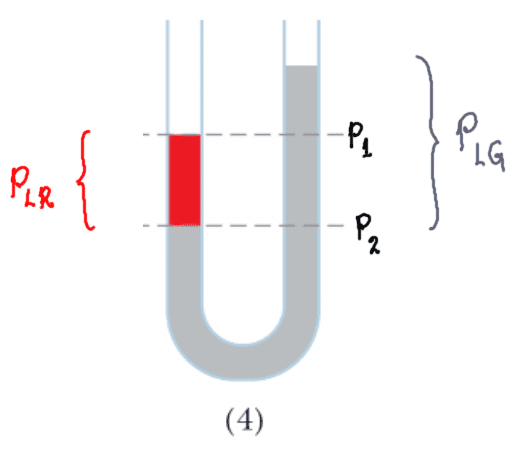Agin the arguments are same as above. Focussing on pressure ##P_2##, we find that the higher level of the grey liquid column must imply that it has a lower density than the red liquid, or ##\boxed{\color{red} {\rho_{LR}} > \color{grey}{\rho_{LG}}}##.